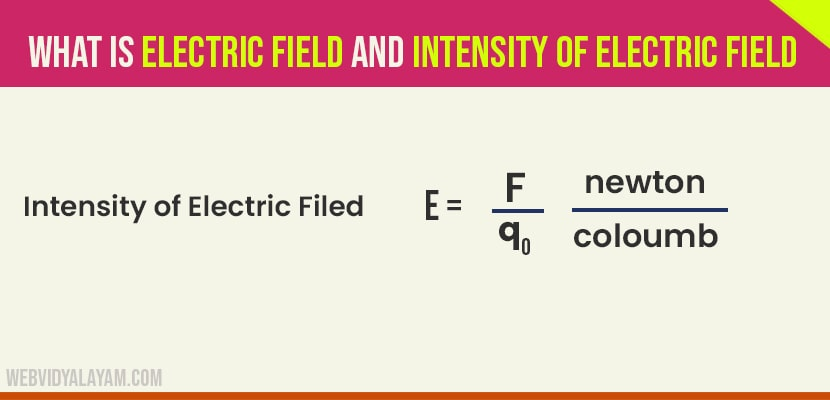# Electric Field and Intensity of Electric Field

## Electric filed

The region surrounding an electric charge or a group of charges, in which another charge experiences a force us called electric filed.

The force between two charges can be considered in terms of electric filed are as follows.

1) The charge q1 sets us an electric filed in space itself.

2) The field of q1 acts on q2, now q2 experiences a force, so the force on q2 is due to the action of field of q1 and q2.

We can also imagine that q2 sets up the field and this field acts on q1, thus produces a force on q1.

Suppose, a receiving antenna is placed in the field of a transmitting antenna. Let at once particular instant, the filed transmitting antenna reaches the receiving antenna. This reaches after a time l/c where l is the separation between two antennas and c is the velocity of light. The filed induces e.m.f in the receiving antenna. The delay in action confirms the intermediatory role of the filed in the forces between the charges.

## Intensity of Electric Field E

The intensity of electric field at a point in the field is defined as the force experienced by a unit positive charge placed at that point.

Let F be the force experienced by a test charge qo placed at a point in the electric filed, then the intensity of electric filed R at that point is given by:

It is obvious that intensity of electric filed is a vector quantity. Its direction will be the same as the direction of force. i.e the direction in which the positive charge placed in the filed tends to move. The unit of electric field is newton per coulomb

Let us consider that a unit positive charge is placed at a distance r from a point charge Q in air. From coulomb’s law, the force on unit positive charge is given by